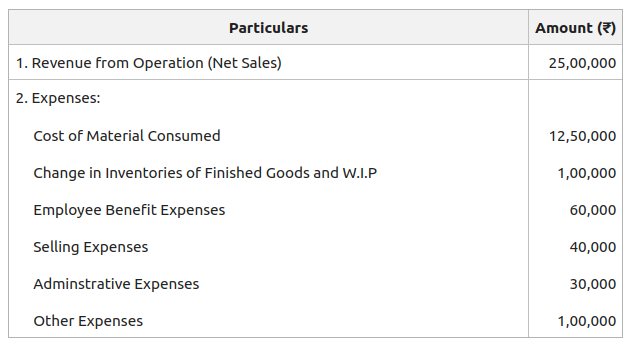Open in App
Not now

# Operating Ratio

• Last Updated : 26 Aug, 2022

The main objective of all the business units is to earn profit. The efficiency of the business can be measured by the profitability of the business. The operating ratio is a type of profitability ratio. It is the comparison of a company’s cost of revenue from the operation and operating expenses to its revenue from operations. Operating Cost (cost of revenue from operation + operating expenses) is the sum total of all the expenses that are incurred in the operating activities of the business. All the non-operating incomes like interest received on investment, profit from the sale of fixed assets, rent received, etc., and all the non-operating expenses like interest on long-term loans and debentures, loss on sale of fixed assets, income tax, dividend paid, etc., are excluded in calculating Operating Ratio.

Operating Ratio =orWhere,

1. Cost of Revenue from Operation (C.O.G.S) = Cost of Material Consumed + Purchase of Stock-in-Trade + Change in Inventories of Finished Goods and Work-in-Progress + Direct Expenses, or

2. Cost of Revenue from Operation (C.O.G.S) = Net Revenue from Operations – Gross Profit

3. Operating Expenses = Employee Benefit Expenses* + Depreciation and Amortisation Expenses + Other Expenses**

*Employee Benefit Expenses include Wages (Direct Expenses) + Salary + Staff Welfare Expenses etc.

**Other Expenses include Selling & Distribution Expenses (Advertisement, Discount, Bad Debts, etc.) + Office and Administration Expenses (Postage, Stationary, Rent, Printing Expenses, Insurance, Repair, etc.)

(Expenses like Loss on Sale of Assets/Investment are included in Other Expenses, but it is a Non-Operating Loss.)

### Significance of Operating Ratio:

The Operational efficiency of the business is measured by operating ratio. The revenue from the operation is compared to the operating cost of the enterprise to know about the percentage of revenue absorbed by the costs. Operating ratio and operating profit ratio are complementary to each other, which means that the lower the operating ratio, the higher the operating profit ratio. Both are calculated in percentage form. A lower operating ratio is considered better. Also, if all the items related to operating costs are studied separately, the management can figure out the areas that require attention.

Illustration 1:

From the following information compute the operating ratio of Geeks Ltd.:Solution:

Cost of Revenue from Operations = Cost of Material Consumed + Change in Inventories of Finished Goods and W.I.P

= 12,50,000 + 1,00,000

= ₹13,50,000

Operating Expenses =  Employee Benefit Expense + Selling Expenses + Administrative Expenses + Other Expenses

= 60,000 + 40,000 + 30,000 + 1,00,000

= 2,30,000

Operating Ratio == 63.2 %

Therefore, Operating Ratio = 63.2 %

Illustration 2:

Using the following information, compute the operating profit ratio for GFG Ltd.:

Revenue from Operations : ₹18,00,000

Operating Expenses : ₹1,80,000

Gross Profit 25% on Cost.

Solution:

Calculation of Cost of Revenue from Operation:

Let Cost of Revenue from Operation be  ₹100

Therefore, Gross Profit =  ₹25, Revenue from Operation = ₹125

If Revenue from Operation is  ₹125, Cost of Revenue from Operation =  ₹100

If Revenue from Operation is  ₹18,00,000,

Cost of Revenue from Operation =Operating Ratio == 90%

Therefore, Operating Ratio = 90 %

My Personal Notes arrow_drop_up
Related Articles